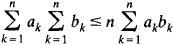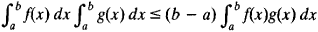# Chebyshev Inequality

Also found in: Dictionary.
Related to Chebyshev Inequality: variance, Markov inequality
The following article is from The Great Soviet Encyclopedia (1979). It might be outdated or ideologically biased.

## Chebyshev Inequality

(1) A basic inequality for monotonic sequences or functions. In the case of the finite sequences a1a2 ≤ . . . ≤ an and blb2 = ≤ . . . ≤ bn it has the formIn integral form we haveHere, f(x) ≥ 0 and g(x) ≥ 0; in addition, the functions are either both increasing or both decreasing. The inequality was derived by P. L. Chebyshev in 1882.

(2) An inequality that provides an estimate of the probability that the deviation of a random variable from its mathematical expectation exceeds some given limit. Let ξ be a random variable, Eξ = a its mathematical expectation, and Dξ = σ2 its variance. The Chebyshev inequality asserts that the probability of the inequality

|ξ – a| ≥ kσ

does not exceed the quantity 1/k2. If ξ is the sum of independent random variables and some additional restrictions are made, then the estimate 1/k2 can be replaced by the estimate 2 exp (–k2/4), which decreases with increasing k much more rapidly.

The inequality is named for P. L. Chebyshev, who used it in 1867 to establish extremely broad conditions for the application of the law of large numbers to sums of independent random variables. (SeeLARGE NUMBERS, LAW OF; LIMIT THEOREMS.)